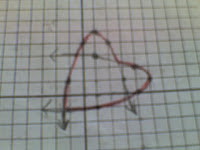## Saturday, May 24, 2008

### I love inverses :)

It's sheer nerd joy, finding the inverse of an exponential or a quadratic function; confirming that entering the output of a relation into its inverse really does return the original input; finding that the graphs of a relation and its inverse really are reflections of each other in the line y = x.I think that requiring all Intermediate Algebra students to do this would be demanding a bit too much, so I offer some worksheets on inverses as extra credit opportunities, and under such conditions many students are more than willing to try. With appropriate enthusiasm, one student highlighted parts of this graph of a quadratic and its inverse in red pencil before turning it in:

I find that this work on inverses deepens students' understanding of the meaning of solving equations, and helps them appreciate the idea that the operations needed to isolate the variable are operations that undo operations previously performed on it. The students need a lot of help on the first examples, and then are quite pleased with themselves when they find they can do this initially hard bit of algebra on their own.

Following Dan Greene, I emphasize the three representations of a relation (Equation! Table! Graph!) again and again, and it is helpful to reiterate these alternative representations when working with inverses. We can find the inverse by interchanging x and y in the equation, by interchanging the values of x and y in the table, or by interchanging the coordinates of each point on the graph of a relation. Talking this way in the context of finding inverses in turn reinforces the idea of equations, tables and graphs as representations of the same information - another nice thing about working with inverses.

Worksheets:
- Exponential and logarithmic functions as inverses, Word and PDF
- Quadratics and square root relations as inverses, Word and PDF